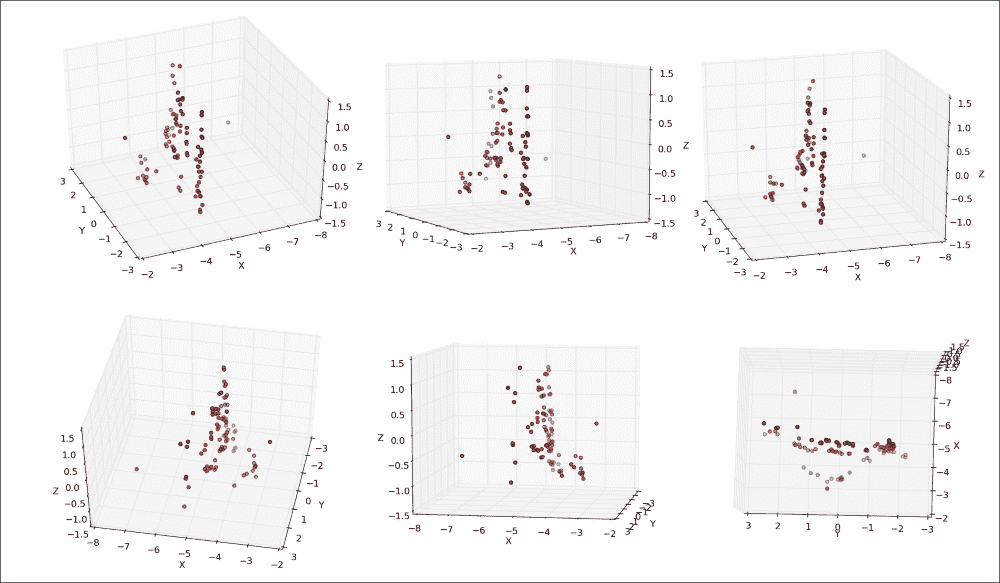# 二十、使用运动结构重建 3D 场景

1. 摄像机校准：我们将使用棋盘图案提取固有的摄像机矩阵以及失真系数，这对于执行场景重建非常重要。
2. 特征匹配：我们将通过加速鲁棒特征SURF）或通过光流来匹配同一视觉场景的两个 2D 图像中的点，如下图所示 ：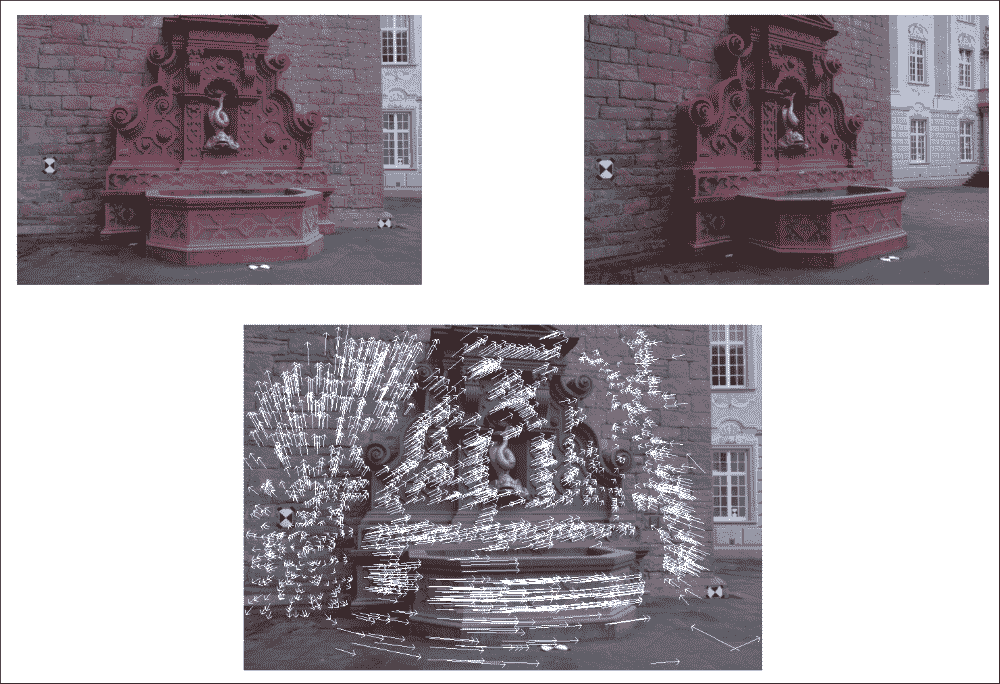3. 图像校正：通过从一对图像中估计摄像机的运动，我们将提取基本矩阵并校正图像。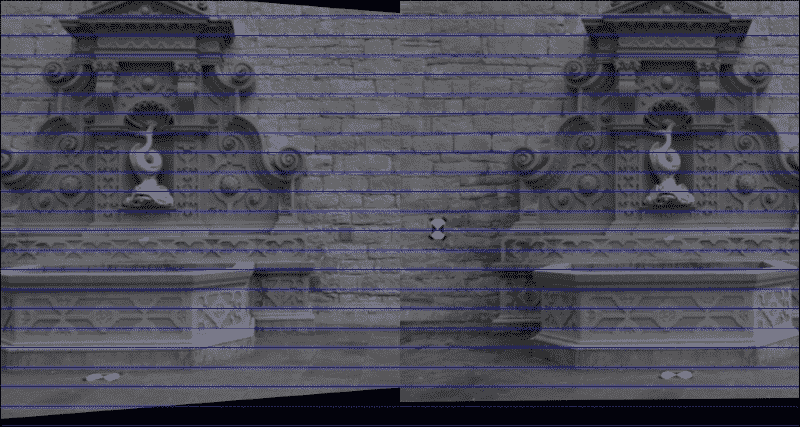4. 三角剖分：我们将利用对极几何形状的约束来重建图像点的 3D 现实世界坐标。

5. 3D 点云可视化：最后，我们将使用 Matplotlib 中的散点图可视化场景的恢复 3D 结构，这在使用pyplot的“平移轴”按钮进行研究时最为引人注目。 使用此按钮可以在所有三个维度上旋转和缩放点云。 如下图所示，在静止帧中的可视化要困难一些（左图：在喷泉的左侧稍稍向前站立，中面板：在喷泉上向下看，右面板：在喷泉中略微站立） 喷泉右前方）：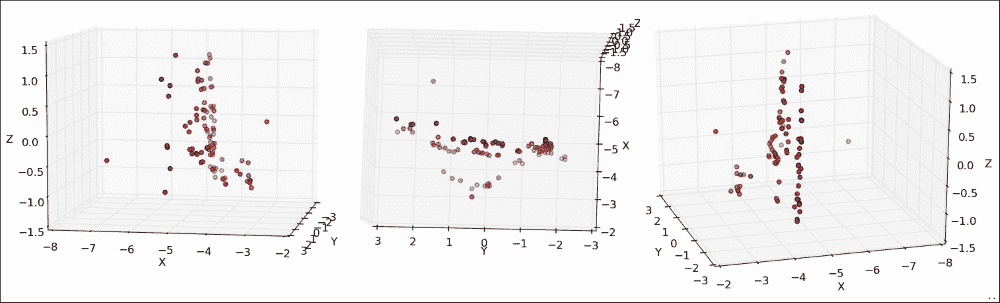# 规划应用

• chapter4.main：这是启动应用的main函数例程。
• scene3D.SceneReconstruction3D：这是一个类，包含用于计算和可视化运动结构的一系列功能。 它包括以下公共方法：
• __init__：此构造器将接受固有的相机矩阵和失真系数
• load_image_pair：一种用于从文件中加载之前描述的相机拍摄的两个图像的方法
• plot_optic_flow：一种用于可视化两个图像帧之间的光流的方法
• draw_epipolar_lines：一种用于绘制两个图像的对极线的方法
• plot_rectified_images：一种用于绘制两个图像的校正版本的方法
• plot_point_cloud：这是一种用于将场景的已恢复现实世界坐标可视化为 3D 点云的方法。 为了到达 3D 点云，我们将需要利用极线几何。 但是，对极几何结构假设使用针孔摄像机模型，因此没有真正的摄像机可以使用。 我们需要校正图像以使其看起来好像来自针孔相机。 为此，我们需要估计摄像机的参数，这将我们引向摄像机校准领域。

# 相机校准

## 针孔相机模型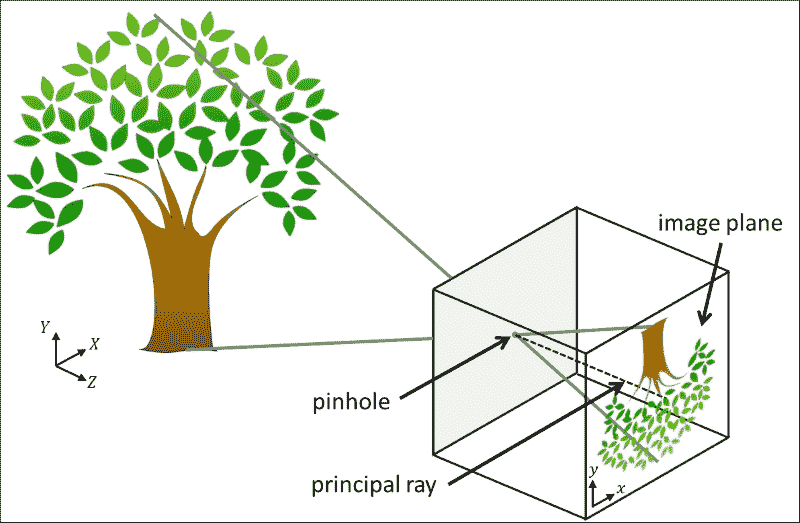Hartley 和 Zisserman 发现了一个数学公式来描述如何从坐标(X, Y, Z)的 3D 点和相机的固有参数推断出具有坐标(x, y)的 2D 点，如下所示：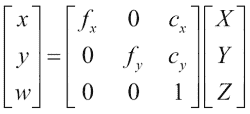## 估计相机的固有参数

import cv2
import numpy as np
import wx

from gui import BaseLayout

def main():
capture = cv2.VideoCapture(0)
if not(capture.isOpened()):
capture.open()

capture.set(cv2.cv.CV_CAP_PROP_FRAME_WIDTH, 640)
capture.set(cv2.cv.CV_CAP_PROP_FRAME_HEIGHT, 480)

# start graphical user interface
app = wx.App()
layout = CameraCalibration(None, -1, 'Camera Calibration', capture)
layout.Show(True)
app.MainLoop()


### 相机校准 GUI

GUI 是通用BaseLayout的定制版本：

class CameraCalibration(BaseLayout):


def _create_custom_layout(self):
"""Creates a horizontal layout with a single button"""
pnl = wx.Panel(self, -1)
self.button_calibrate = wx.Button(pnl,label='Calibrate Camera')
self.Bind(wx.EVT_BUTTON, self._on_button_calibrate)
hbox = wx.BoxSizer(wx.HORIZONTAL)
pnl.SetSizer(hbox)


self.panels_vertical.Add(pnl, flag=wx.EXPAND | wx.BOTTOM | wx.TOP, border=1)


### 初始化算法

def _init_custom_layout(self):
"""Initializes camera calibration"""
# setting chessboard size
self.chessboard_size = (9, 6)


# prepare object points
self.objp = np.zeros((np.prod(self.chessboard_size), 3), dtype=np.float32)
self.objp[:, :2] = np.mgrid[0:self.chessboard_size,0:self.chessboard_size].T.reshape(-1, 2)


    # prepare recording
self.recording = False
self.record_min_num_frames = 20
self._reset_recording()


def _on_button_calibrate(self, event):
self.button_calibrate.Disable()
self.recording = True
self._reset_recording()


def _reset_recording(self):
self.record_cnt = 0
self.obj_points = []
self.img_points = []


### 收集图像和对象点

_process_frame方法由负责完成校准技术的艰苦工作。 单击self.button_calibrate按钮后，此方法开始收集数据，直到检测到总共self.record_min_num_frames个棋盘为止：

def _process_frame(self, frame):
"""Processes each frame"""

# if we are not recording, just display the frame
if not self.recording:
return frame

# else we're recording
img_gray = cv2.cvtColor(frame, cv2.COLOR_BGR2GRAY).astype(np.uint8)

if self.record_cnt < self.record_min_num_frames:
ret, corners = cv2.findChessboardCorners(img_gray, self.chessboard_size, None)


cv2.findChessboardCorners函数将解析灰度图像（img_gray）以找到大小为self.chessboard_size的棋盘。 如果图像确实包含棋盘，该函数将返回trueret）以及棋盘角列表（corners）。

if ret:
cv2.drawChessboardCorners(frame, self.chessboard_size, corners, ret)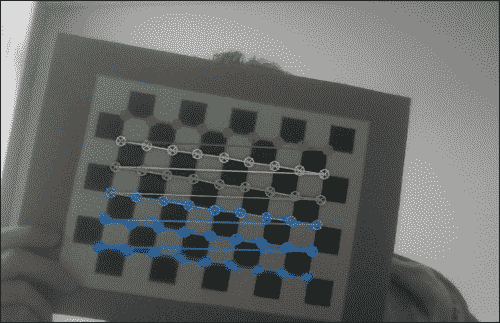criteria = (cv2.TERM_CRITERIA_EPS +
cv2.TERM_CRITERIA_MAX_ITER, 30, 0.01)
cv2.cornerSubPix(img_gray, corners, (9, 9), (-1, -1),
criteria)


self.obj_points.append(self.objp)
self.img_points.append(corners)
self.record_cnt += 1


### 查找相机矩阵

else:
print "Calibrating..."
ret, K, dist, rvecs, tvecs = cv2.calibrateCamera(self.obj_points, self.img_points, (self.imgHeight, self.imgWidth), None, None)


print "K=", K
print "dist=", dist


K= [[ 3.36696445e+03 0.00000000e+00 2.99109943e+02]
[ 0.00000000e+00 3.29683922e+03 2.69436829e+02]
[ 0.00000000e+00 0.00000000e+00 1.00000000e+00]]
dist= [[ 9.87991355e-01 -3.18446968e+02 9.56790602e-02 -3.42530800e-02 4.87489304e+03]]


mean_error = 0
for i in xrange(len(self.obj_points)):
img_points2, _ = cv2.projectPoints(self.obj_points[i], rvecs[i], tvecs[i], K, dist)
error = cv2.norm(self.img_points[i], img_points2, cv2.NORM_L2)/len(img_points2)
mean_error += error

print "mean error=",  {} pixels".format(mean_error)


# 设置应用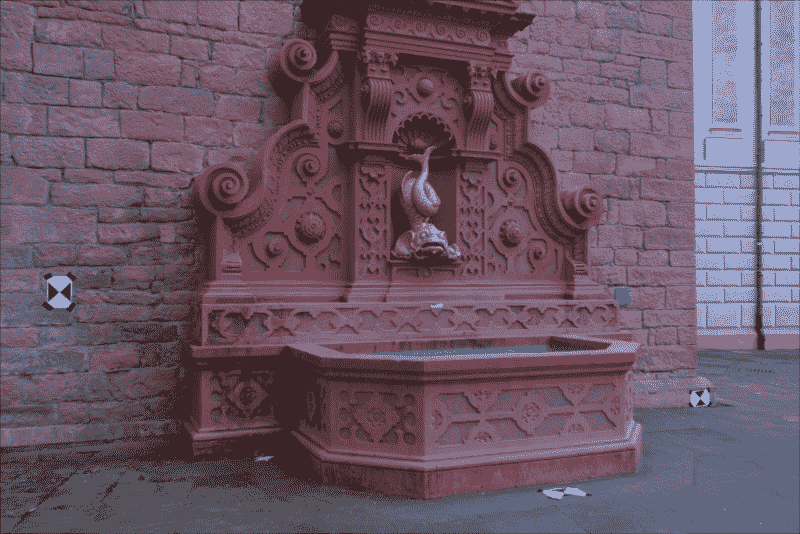## main函数例程

import numpy as np

from scene3D import SceneReconstruction3D

def main():
# camera matrix and distortion coefficients
# can be recovered with calibrate.py
# but the examples used here are already undistorted, taken
# with a camera of known K
K = np.array([[2759.48/4, 0, 1520.69/4, 0, 2764.16/4,1006.81/4, 0, 0, 1]]).reshape(3, 3)
d = np.array([0.0, 0.0, 0.0, 0.0, 0.0]).reshape(1, 5)


### 注意

# load a pair of images for which to perform SfM
scene = SceneReconstruction3D(K, d)


scene.plot_optic_flow()
scene.draw_epipolar_lines()
scene.plot_rectified_images()

# draw 3D point cloud of fountain
# use "pan axes" button in pyplot to inspect the cloud (rotate
# and zoom to convince you of the result)
scene.plot_point_cloud()


## SceneReconstruction3D类

import cv2
import numpy as np
import sys

from mpl_toolkits.mplot3d import Axes3D
import matplotlib.pyplot as plt

class SceneReconstruction3D:
def __init__(self, K, dist):
self.K = K
self.K_inv = np.linalg.inv(K)
self.d = dist


def load_image_pair(self, img_path1, img_path2,downscale=True):

# make sure images are valid
if self.img1 is None:
sys.exit("Image " + img_path1 + " could not be loaded.")
if self.img2 is None:
sys.exit("Image " + img_path2 + " could not be loaded.")


if len(self.img1.shape)==2:
self.img1 = cv2.cvtColor(self.img1, cv2.COLOR_GRAY2BGR)
self.img2 = cv2.cvtColor(self.img2, cv2.COLOR_GRAY2BGR)


# scale down image if necessary
# to something close to 600px wide
target_width = 600
if downscale and self.img1.shape>target_width:
while self.img1.shape > 2*target_width:
self.img1 = cv2.pyrDown(self.img1)
self.img2 = cv2.pyrDown(self.img2)


self.img1 = cv2.undistort(self.img1, self.K, self.d)
self.img2 = cv2.undistort(self.img2, self.K, self.d)


# 从一对图像估计相机运动

def ___extract_keypoints(self, feat_mode):
if featMode == "SURF":
# feature matching via SURF and BFMatcher
self._extract_keypoints_surf()
else:
if feat_mode == "flow":
# feature matching via optic flow
self._extract_keypoints_flow()
else:
sys.exit("Unknown mode " + feat_mode+ ". Use 'SURF' or 'FLOW'")


## 使用丰富特征描述符的点匹配

def _extract_keypoints_surf(self):
detector = cv2.SURF(250)
first_key_points, first_des = detector.detectAndCompute(self.img1, None)
second_key_points, second_desc = detector.detectAndCompute(self.img2, None)


matcher = cv2.BFMatcher(cv2.NORM_L1, True)
matches = matcher.match(first_desc, second_desc)


first_match_points = np.zeros((len(matches), 2), dtype=np.float32)
second_match_points = np.zeros_like(first_match_points)
for i in range(len(matches)):
first_match_points[i] =
first_key_points[matches[i].queryIdx].pt
second_match_points[i] =
second_key_points[matches[i].trainIdx].pt

self.match_pts1 = first_match_points
self.match_pts2 = second_match_points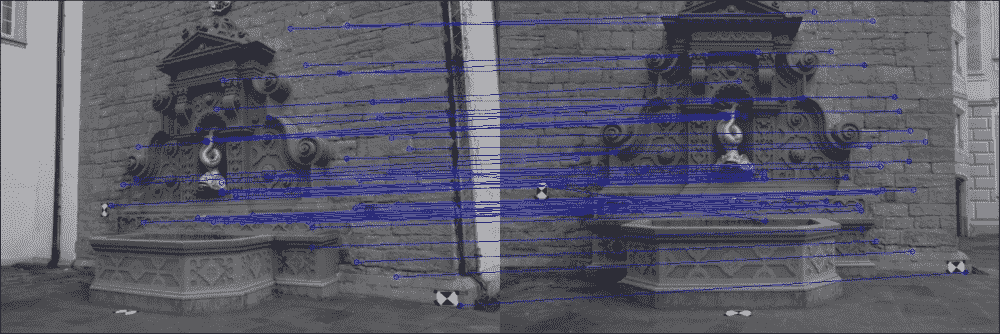## 使用光流的点匹配

Lukas-Kanade 方法是计算密集光通量最常用的技术之一。 通过使用cv2.calcOpticalFlowPyrLK函数，可以用一行代码在 OpenCV 中实现。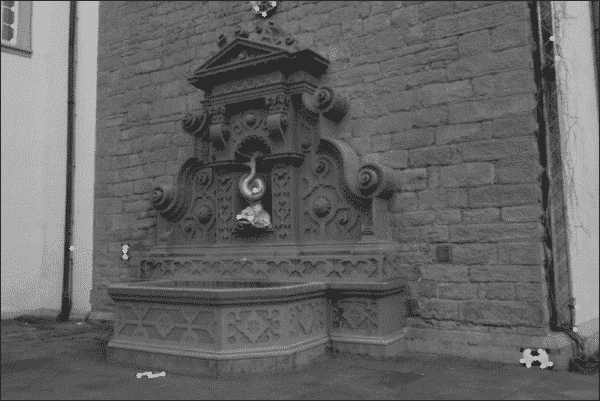def _extract_keypoints_flow(self):
fast = cv2.FastFeatureDetector()
first_key_points = fast.detect(self.img1, None)


first_key_list = [i.pt for i in first_key_points]
first_key_arr = np.array(first_key_list).astype(np.float32)


second_key_arr, status, err = cv2.calcOpticalFlowPyrLK(self.img1, self.img2, first_key_arr)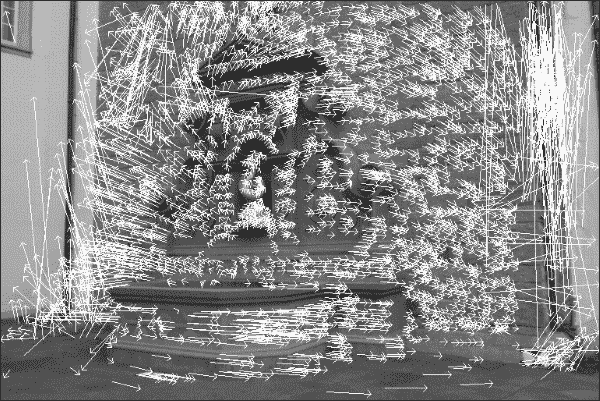condition = (status == 1) * (err < 5.)
concat = np.concatenate((condition, condition), axis=1)
first_match_points = first_key_arr[concat].reshape(-1, 2)
second_match_points = second_key_arr[concat].reshape(-1, 2)

self.match_pts1 = first_match_points
self.match_pts2 = second_match_points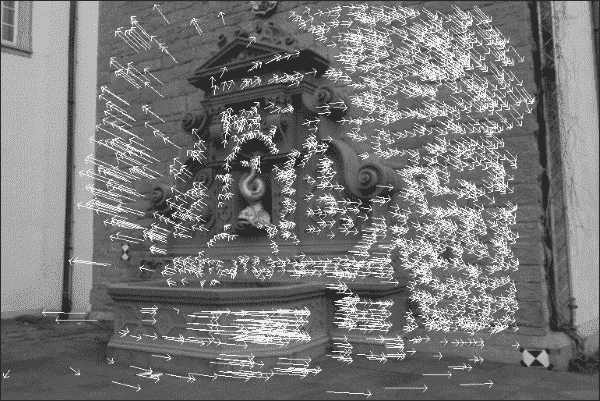def plot_optic_flow(self):
self._extract_key_points("flow")

img = self.img1
for i in xrange(len(self.match_pts1)):
cv2.line(img, tuple(self.match_pts1[i]), tuple(self.match_pts2[i]), color=(255, 0, 0))
theta = np.arctan2(self.match_pts2[i] – self.match_pts1[i], self.match_pts2[i] – self.match_pts1[i])
cv2.line(img, tuple(self.match_pts2[i]),
(np.int(self.match_pts2[i] – 6*np.cos(theta+np.pi/4)),
np.int(self.match_pts2[i] –
6*np.sin(theta+np.pi/4))), color=(255, 0, 0))
cv2.line(img, tuple(self.match_pts2[i]), (np.int(self.match_pts2[i] –6*np.cos(theta-np.pi/4)),np.int(self.match_pts2[i] –
6*np.sin(theta-np.pi/4))), color=(255, 0, 0))
for i in xrange(len(self.match_pts1)):
cv2.line(img, tuple(self.match_pts1[i]), tuple(self.match_pts2[i]), color=(255, 0, 0))
theta = np.arctan2(self.match_pts2[i] -self.match_pts1[i],self.match_pts2[i] - self.match_pts1[i])
cv2.imshow("imgFlow",img)
cv2.waitKey()


## 查找相机矩阵

def _find_fundamental_matrix(self):
self.F, self.Fmask = cv2.findFundamentalMat(self.match_pts1, self.match_pts2, cv2.FM_RANSAC, 0.1, 0.99)


def _find_essential_matrix(self):
self.E = self.K.T.dot(self.F).dot(self.K)


def _find_camera_matrices(self):
U, S, Vt = np.linalg.svd(self.E)
W = np.array([0.0, -1.0, 0.0, 1.0, 0.0, 0.0, 0.0, 0.0, 1.0]).reshape(3, 3)


first_inliers = []
second_inliers = []
first_inliers.append(self.K_inv.dot([self.match_pts1[i], self.match_pts1[i], 1.0]))
second_inliers.append(self.K_inv.dot([self.match_pts2[i], self.match_pts2[i], 1.0]))


# First choice: R = U * Wt * Vt, T = +u_3 (See Hartley
# & Zisserman 9.19)
R = U.dot(W).dot(Vt)
T = U[:, 2]

if not self._in_front_of_both_cameras(first_inliers,second_inliers, R, T):
# Second choice: R = U * W * Vt, T = -u_3
T = - U[:, 2]

if not self._in_front_of_both_cameras(first_inliers, second_inliers, R, T):
# Third choice: R = U * Wt * Vt, T = u_3
R = U.dot(W.T).dot(Vt)
T = U[:, 2]

if not self._in_front_of_both_cameras(first_inliers, second_inliers, R, T):
# Fourth choice: R = U * Wt * Vt, T = -u_3
T = - U[:, 2]


self.Rt1 = np.hstack((np.eye(3), np.zeros((3, 1))))


self.Rt2 = np.hstack((R, T.reshape(3, 1)))


__InFrontOfBothCameras私有方法是一个辅助函数，可确保将每对关键点映射到 3D 坐标，使它们位于两个摄像机的前面：

def _in_front_of_both_cameras(self, first_points, second_points, rot, trans):
rot_inv = rot
for first, second in zip(first_points, second_points):
first_z = np.dot(rot[0, :] - second*rot[2, :], trans) / np.dot(rot[0, :] - second*rot[2, :], second)
first_3d_point = np.array([first * first_z, second * first_z, first_z])
second_3d_point = np.dot(rot.T, first_3d_point) – np.dot(rot.T, trans)


if first_3d_point < 0 or second_3d_point < 0:
return False
return True


## 图像校正

def plot_rectified_images(self, feat_mode="SURF"):
self._extract_keypoints(feat_mode)
self._find_fundamental_matrix()
self._find_essential_matrix()
self._find_camera_matrices_rt()

R = self.Rt2[:, :3]
T = self.Rt2[:, 3]


    R1, R2, P1, P2, Q, roi1, roi2 = cv2.stereoRectify(self.K, self.d, self.K, self.d, self.img1.shape[:2], R, T,
alpha=1.0)
mapx1, mapy1 = cv2.initUndistortRectifyMap(self.K, self.d, R1, self.K, self.img1.shape[:2], cv2.CV_32F)
mapx2, mapy2 = cv2.initUndistortRectifyMap(self.K, self.d, R2, self.K, self.img2.shape[:2], cv2.CV_32F)
img_rect1 = cv2.remap(self.img1, mapx1, mapy1, cv2.INTER_LINEAR)
img_rect2 = cv2.remap(self.img2, mapx2, mapy2, cv2.INTER_LINEAR)


total_size = (max(img_rect1.shape, img_rect2.shape), img_rect1.shape + img_rect2.shape, 3)
img = np.zeros(total_size, dtype=np.uint8)
img[:img_rect1.shape, :img_rect1.shape] = img_rect1
img[:img_rect2.shape, img_rect1.shape:] = img_rect2


for i in range(20, img.shape, 25):
cv2.line(img, (0, i), (img.shape, i), (255, 0, 0))
cv2.imshow('imgRectified', img)# 重建场景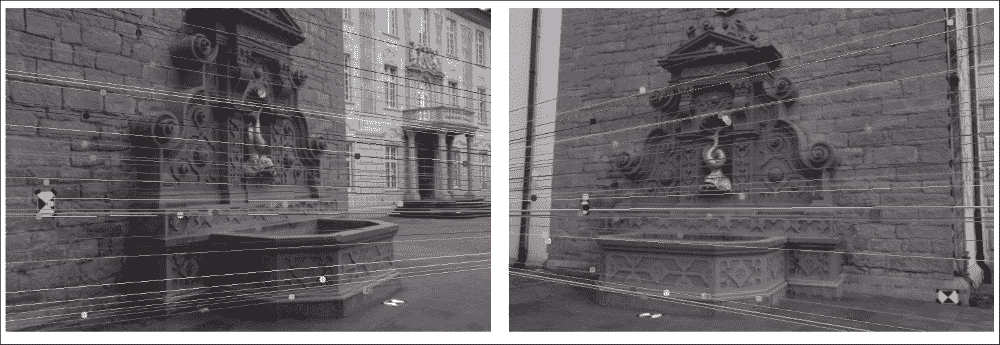first_inliers = np.array(self.match_inliers1).reshape(-1, 3)[:, :2]
second_inliers = np.array(self.match_inliers2).reshape(-1, 3)[:, :2]


pts4D = cv2.triangulatePoints(self.Rt1, self.Rt2, first_inliers.T,second_inliers.T).T


pts3D = pts4D[:, :3]/np.repeat(pts4D[:, 3], 3).reshape(-1, 3)


# 3D 点云可视化

def plot_point_cloud(self, feat_mode="SURF"):
self._extract_keypoints(feat_mode)
self._find_fundamental_matrix()
self._find_essential_matrix()
self._find_camera_matrices_rt()

# triangulate points
first_inliers = np.array(self.match_inliers1).reshape(-1, 3)[:, :2]
second_inliers = np.array(self.match_inliers2).reshape(-1, 3)[:, :2]
pts4D = cv2.triangulatePoints(self.Rt1, self.Rt2,first_inliers.T, second_inliers.T).T

# convert from homogeneous coordinates to 3D
pts3D = pts4D[:, :3]/np.repeat(pts4D[:, 3], 3).reshape(-1, 3)


    Ys = pts3D[:,0]
Zs = pts3D[:,1]
Xs = pts3D[:,2]

fig = plt.figure()
ax.scatter(Xs, Ys, Zs, c='r', marker='o')
ax.set_xlabel('Y')
ax.set_ylabel('Z')
ax.set_zlabel('X')
plt.show()